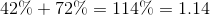## Example Questions

### Example Question #1 : Decimals And Percentage

Students at a country fair typically spend $40. The students have agreed to spend 40% less at this year's fair. Assuming all the students follow their new agreement, what is the most that could be spent by a student at this year's fair? Possible Answers:$16

$10$30

$24$20

$24 Explanation: This is a percentage problem, so it is important to know that 40% of some value is equal to 0.4 of said value. So to find 40% of$40, we multiply 40 * 0.40, which equals 16.  This 16 represents 40% of the original value of $40. So if a student was to spend 40% less than they usually spend, we would subtract$16, which is 40% of the original value, from $40, giving us an answer of$24.

### Example Question #2 : Decimals And Percentage

There are n pennies in a jar. Let p represent the decimal ratio of the pennies that are dated before 1983. Which of the following equations repesents the number of pennies that are dated 1983 or later?

n(100-p)

np

n(1-p)

n(1-p)/100

n(1-p)

Explanation:

The proportion of pennies before 1983 would be np. Therefore, the pennies dated 1983 or later would be n(1-p).

### Example Question #3 : Decimals And Percentage

Express the following as a decimal: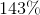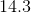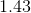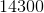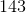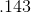Explanation:

To convert a percentage to a decimal, you must add a decimal two points to the left from the right hand side. Thus,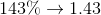### Example Question #4 : Decimals And Percentage

Which of the following is equivalent to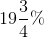?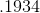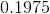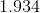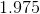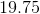Explanation:

To find a decimal from a percentage, divide the percentage value by. In this case, convert the fraction to a decimal first: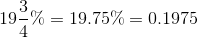### Example Question #5 : Decimals And Percentage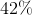share in a stock is added to a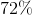share by speculators. What is the decimal equivalent of the combined shares?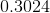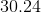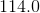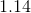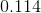To convert from a percentage to a decimal, divide the decimal by. In this case, we can add the percentages first.